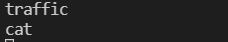# Pass the dropdown selected value to NodeJS for querying database

i have created one dropdown that passes data to NodeJS through axios post i can see that in console log of nodejs Post but cant able to use the value outside the post function

i want to use the value to querying the database

my nodejs code:

```  app.post('/getmodel', (req, res) => {
var model = req.body.model;

console.log(model);
//It shows model value here but can't able to use outside

});

app.get('/model', (req,res)=>{
let model = req.body.model;
let sql ="select * from new_schema.model_list,new_schema.images where model_name=  " + mysql.escape(model)
db.query(sql,model, (err,results) =>{
if(err){
throw err
}
console.log(results)
res.send(results);
})
})```

my react code works fine as i can able to see the selected value in nodejs console belowthese are the selected value from my dropdown that shows in my nodejs console. but in cant use it by req.body like that please help me

Contents

### Method 1

You can try like this

```app.post('/getmodel', (req, res) => {
var model = req.body.model;

console.log(model);
//It shows model value here and you can able to use in the query

let sql = "select * from new_schema.model_list,new_schema.images where model_name=  " + mysql.escape(model)
db.query(sql, model, (err, results) => {
if (err) {
throw err
}
console.log(results)
res.send(results);
})

});```